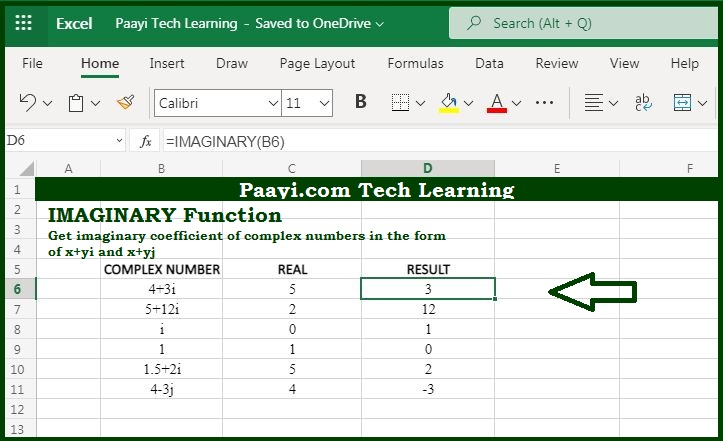# Learn How to Use Microsoft Excel IMAGINARY Function

Written by | 0 Comments | 668 Views

In this article, you will learn how to use the Microsoft Excel IMAGINARY function and its prime function in Microsoft Excel. You will also get to know the Microsoft Excel IMAGINARY function return value and syntax with the help of some examples.

Microsoft Excel IMAGINARY Function

The prime function of the Microsoft Excel IMAGINARY function is to get the imaginary coefficient of the complex number. So, with the help of the IMAGINARY function, you can able to return the imaginary coefficient of the complex number in the form of  "x + Yi" or "x + yj".

Return Value of IMAGINARY Function

The return value will be the imaginary coefficient of the complex number.

Syntax of IMAGINARY Function

=IMAGINARY(inumber)

Where the arguments:

inumber: It is a complex number.

## How to Use Microsoft Excel IMAGINARY Function?As we know that IMAGINARY function you can able to return the imaginary coefficient of the complex number in the form of  "x + yi" or "x + yj". You can also use the COMPLEX function to create a complex number from real and imaginary parts.

It should be noted that only lowercase "i" and "j" is accepted by the IAMBS function and other values will result in a #NUM! error.